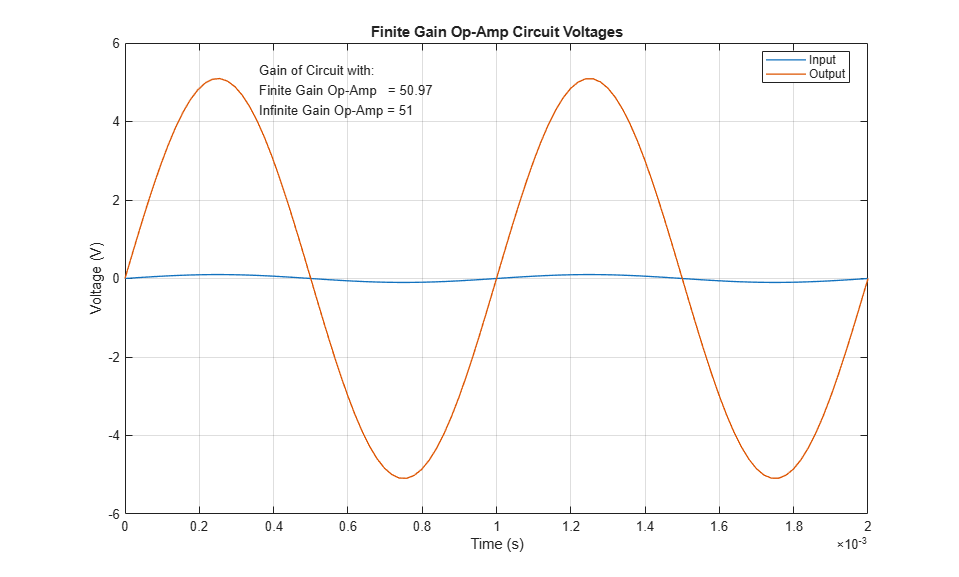# Finite-Gain Op-Amp

This example shows how higher fidelity or more detailed component models can be built from the Foundation library blocks. The Op-Amp block in the Foundation library models the ideal case whereby the gain is infinite, input impedance infinite, and output impedance zero. The Finite Gain Op-Amp block in this example has an open-loop gain of 1e5, input resistance of 100K ohms and output resistance of 10 ohms. As a result, the gain for this amplifier circuit is slightly lower than the gain that can be analytically calculated if the op-amp gain is assumed to be infinite.

### Model### Finite Gain Op-Amp Subsystem### Simulation Results from Simscape Logging

Plot "Finite Gain Op-Amp Circuit Voltages" shows the input and output voltages for the circuit. If the circuit used an infinite gain op-amp with no input and output resistances defined, the gain would be 1+R2/R1 = 51. Since this model uses an op-amp with finite gain plus input and output resistances, the circuit gain is slightly less.